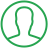# Fibonacci Series In Java Using Recursion

Last Updated : Jul 1, 2023In this article we will show you the solution of Fibonacci series in java using recursion, a Fibonacci series consists of numbers that are the sum of their previous two numbers (except for the first two). There are usually 2 and 0 at the beginning of a Fibonacci series.

The Fibonacci series can be created using recursion or iteration in Java.

From the Fibonacci sequence, the Wythoff array gives rise to an infinite matrix of numbers.

Fibonacci is a set of numbers with the next number equaling the sum of the two previous numbers. Fibonacci series begins with zero and one, the first two numbers on the series.

To write a Java program using the fibonacci series, there are two ways:

• An analysis of Fibonacci sequences without recursion
• Using recursion, we can calculate the Fibonacci Series

A Fibonacci series begins with 0 and consists of 2 terms, with the rest equal to (n-1)th and (n-2)th terms.

Example: 0, 1, 1, 2, 3, 5, 8, 13, 21, 34, 55, 89 …

### Method - Use of loops

Calculate the number of terms in Fibonacci series by putting in the value of n.

In this case, a=0, b=1, and c=0 are assigned to the first element, second element, and third element, respectively.

Loop to generate the next two elements after the first two elements have been output.

Output the value of c by updating the variable as c=a+b, a=b, and b=c.

Since all of the words have already been formed, the process of producing them is restarted.

By observing the recursive nature of this method, we will discover that we have been recalculating the series for each term.

As a result of the overhead of the recalculation, this program's time complexity increases to O(n2).

By using dynamic programming, this recursive algorithm can be made more efficient.

## Step By Step Guide On Fibonacci Series In Java Using Recursion :-

```import java.io.*;
class Fibonacci recursion {
static int fib(int n)
{
if (n <= 1)
return n;
return fib(n - 1) + fib(n - 2);
}
public static void main(String args[])
{
int n = 10;
System.out.println(fib(n));
}
}```
1. First we create an import function with the name java.io.*
2. In the next step, we create a class called Fibonacci recursion.
3. next step is to create a static function
4. Our next step is to describe series by using the if and return functions.
5. As a result, a public static void main is created as a string argument.
6. Our next step is to create an integer number.
7. After that we close program using system.out.println.

## Conclusion :-

Java's Fibonacci Series is a series of numbers with each number equal to the sum of its two neighbours.

In the Fibonacci series, the first two numbers are zero and one. An algorithm that determines the greatest common divisor of two integers is heavily dependent on the Fibonacci numbers.

I hope this article on Fibonacci series in java using recursion helps you and the steps and method mentioned above are easy to follow and implement.

##About Dikshita

Passionate Electronics and Communication Engineering student with expertise in web development (HTML, CSS, JS,PHP, Bootstrap, React.js) and content writing. Eager problem solver and tech enthusiast, adept at creating engaging web experiences.